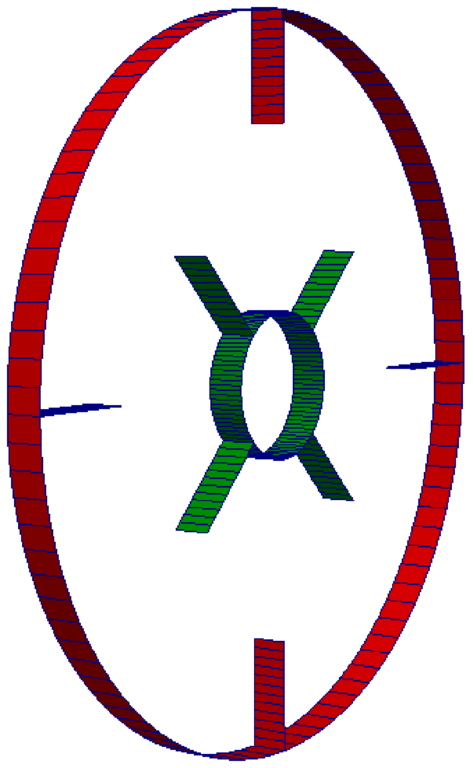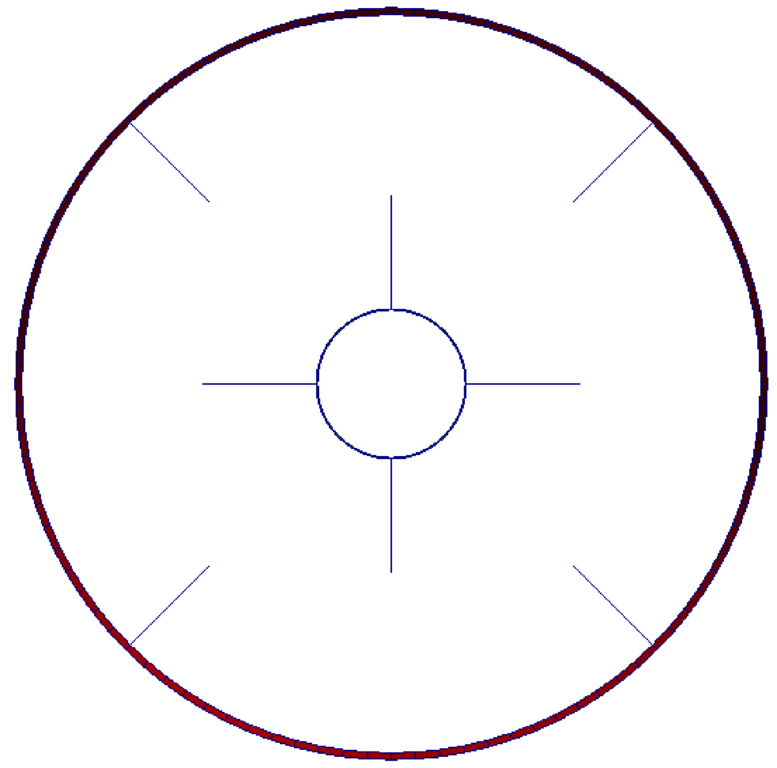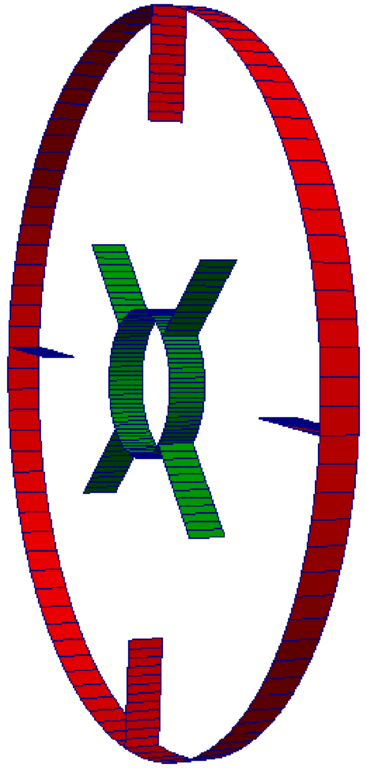Previous: Basic test case Up: Basic test case Next: Test case set up
This is an automatically generated documentation by LaTeX2HTML utility. In case of any issue, please, contact us at info@cfdsupport.com.

## Geometry description

• In this test case we assume an idealized model of mixer in two dimensions. The test case will be later extended to three dimensions and modified.
• Basic test case geometry is following:Figure: OpenFOAM tutorial mixerVessel2D. Geometry view.

• The test case is two-dimensional
• In the center of the domain there is a rotor with four blades
• Peripheral wall is not moving
• Steady-state solution of turbulent flow of incompressible fluid
• For rotation Multiple Reference Frame (MRF) method is used
• MRF adds source term (acceleration) to velocity (momentum) equations
• Source term is used on volume cells cellZone